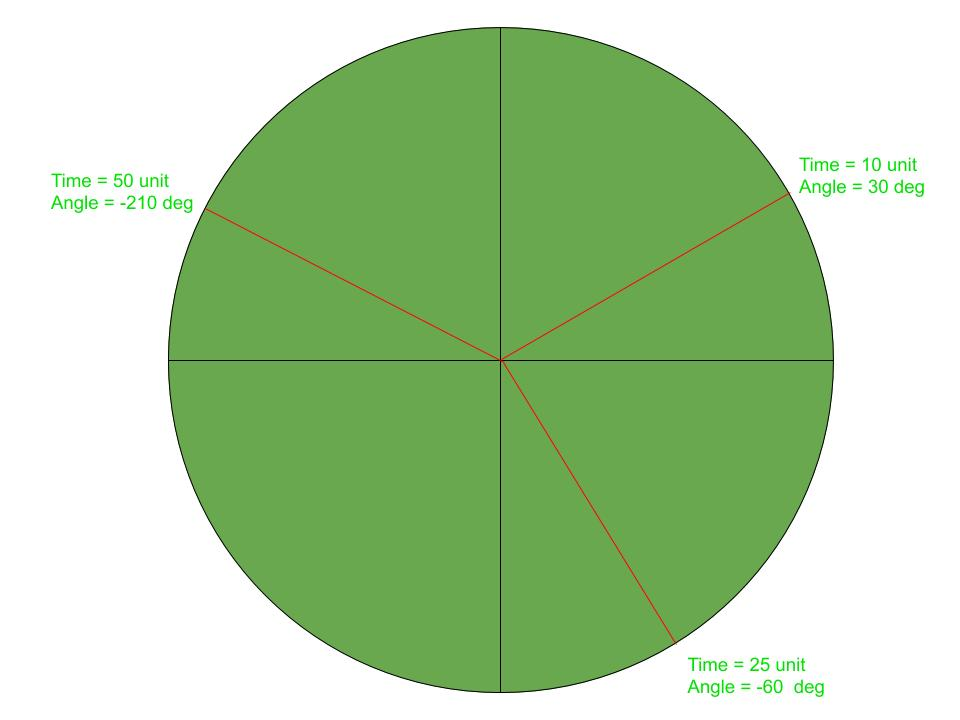GFG App
Open AppBrowser
Continue

# Java Applet | How to display an Analog Clock

In this article, we shall be animating the applet window to show an Analog Clock with a 1-second delay. The idea is to display the system time of every instance.Approach:
Each hand of the clock will be animating with 1-second delay keeping one end at the centre. The position of the other end can be derived by the system time. The angle formed by a hand of the clock in every second will be different throughout its journey. This is why various instances make a different angle to the horizontal line.

How to run:

```1. Save the file as analogClock.java
and run the following commands.
2. javac analogClock.java
3. appletviewer analogClock.java
```

Below is the implementation of the above approach:

Program:

 `// Java program to illustrate ` `// analog clock using Applets ` ` `  `import` `java.applet.Applet; ` `import` `java.awt.*; ` `import` `java.util.*; ` ` `  `public` `class` `analogClock ``extends` `Applet { ` ` `  `    ``@Override` `    ``public` `void` `init() ` `    ``{ ` `        ``// Applet window size & color ` `        ``this``.setSize(``new` `Dimension(``800``, ``400``)); ` `        ``setBackground(``new` `Color(``50``, ``50``, ``50``)); ` `        ``new` `Thread() { ` `            ``@Override` `            ``public` `void` `run() ` `            ``{ ` `                ``while` `(``true``) { ` `                    ``repaint(); ` `                    ``delayAnimation(); ` `                ``} ` `            ``} ` `        ``}.start(); ` `    ``} ` ` `  `    ``// Animating the applet ` `    ``private` `void` `delayAnimation() ` `    ``{ ` `        ``try` `{ ` ` `  `            ``// Animation delay is 1000 milliseconds ` `            ``Thread.sleep(``1000``); ` `        ``} ` `        ``catch` `(InterruptedException e) { ` `            ``e.printStackTrace(); ` `        ``} ` `    ``} ` ` `  `    ``// Paint the applet ` `    ``@Override` `    ``public` `void` `paint(Graphics g) ` `    ``{ ` `        ``// Get the system time ` `        ``Calendar time = Calendar.getInstance(); ` ` `  `        ``int` `hour = time.get(Calendar.HOUR_OF_DAY); ` `        ``int` `minute = time.get(Calendar.MINUTE); ` `        ``int` `second = time.get(Calendar.SECOND); ` ` `  `        ``// 12 hour format ` `        ``if` `(hour > ``12``) { ` `            ``hour -= ``12``; ` `        ``} ` ` `  `        ``// Draw clock body center at (400, 200) ` `        ``g.setColor(Color.white); ` `        ``g.fillOval(``300``, ``100``, ``200``, ``200``); ` ` `  `        ``// Labeling ` `        ``g.setColor(Color.black); ` `        ``g.drawString(``"12"``, ``390``, ``120``); ` `        ``g.drawString(``"9"``, ``310``, ``200``); ` `        ``g.drawString(``"6"``, ``400``, ``290``); ` `        ``g.drawString(``"3"``, ``480``, ``200``); ` ` `  `        ``// Declaring variables to be used ` `        ``double` `angle; ` `        ``int` `x, y; ` ` `  `        ``// Second hand's angle in Radian ` `        ``angle = Math.toRadians((``15` `- second) * ``6``); ` ` `  `        ``// Position of the second hand ` `        ``// with length 100 unit ` `        ``x = (``int``)(Math.cos(angle) * ``100``); ` `        ``y = (``int``)(Math.sin(angle) * ``100``); ` ` `  `        ``// Red color second hand ` `        ``g.setColor(Color.red); ` `        ``g.drawLine(``400``, ``200``, ``400` `+ x, ``200` `- y); ` ` `  `        ``// Minute hand's angle in Radian ` `        ``angle = Math.toRadians((``15` `- minute) * ``6``); ` ` `  `        ``// Position of the minute hand ` `        ``// with length 80 unit ` `        ``x = (``int``)(Math.cos(angle) * ``80``); ` `        ``y = (``int``)(Math.sin(angle) * ``80``); ` ` `  `        ``// blue color Minute hand ` `        ``g.setColor(Color.blue); ` `        ``g.drawLine(``400``, ``200``, ``400` `+ x, ``200` `- y); ` ` `  `        ``// Hour hand's angle in Radian ` `        ``angle = Math.toRadians((``15` `- (hour * ``5``)) * ``6``); ` ` `  `        ``// Position of the hour hand ` `        ``// with length 50 unit ` `        ``x = (``int``)(Math.cos(angle) * ``50``); ` `        ``y = (``int``)(Math.sin(angle) * ``50``); ` ` `  `        ``// Black color hour hand ` `        ``g.setColor(Color.black); ` `        ``g.drawLine(``400``, ``200``, ``400` `+ x, ``200` `- y); ` `    ``} ` `} `

Output:

My Personal Notes arrow_drop_up